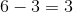# Pre-Algebra : Negative Numbers

## Example Questions

### Example Question #11 : Subtract Rational Numbers And Understand The Absolute Value Of Their Difference: Ccss.Math.Content.7.Ns.A.1c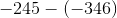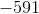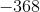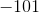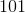Explanation:Two negative signs become a plus sign. Since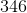is greater than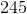and is positive, our answer is positive. We treat as a normal subtraction.

Answer is### Example Question #12 : Subtract Rational Numbers And Understand The Absolute Value Of Their Difference: Ccss.Math.Content.7.Ns.A.1c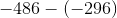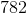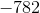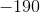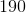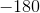Explanation:Two minus signs become a plus sign. Since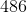is greater than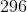and is negative, our answer is negative. We treat as a normal subtraction.

Answer is.

### Example Question #1 : Interpret Products Of Rational Numbers And Understand Properites Of Operations: Ccss.Math.Content.7.Ns.A.2a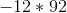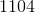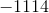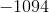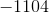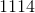Explanation:When multiplying with a negative number, our answer is negative.

Answer is### Example Question #10 : Interpret Products Of Rational Numbers And Understand Properites Of Operations: Ccss.Math.Content.7.Ns.A.2a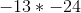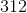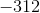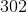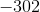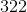Explanation:When multiplying with two negative numbers, our answer is positive.

Answer is### Example Question #83 : Negative Numbers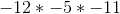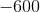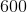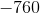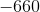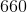Explanation:When there are odd amount of negative numbers, the answer is negative when multiplied. Since three is odd, our answer is negative. We multiply from left to right.

Answer is.

### Example Question #7 : Divide Integers: Ccss.Math.Content.7.Ns.A.2b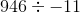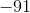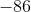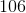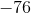Explanation:When dividing with a negative number, our answer is negative.

Answer is.

### Example Question #1 : Divide Integers: Ccss.Math.Content.7.Ns.A.2b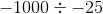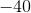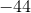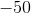Explanation:When dividing with two negative numbers, our answer is positive.

Answer is### Example Question #84 : Negative Numbers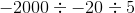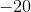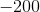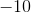Explanation:When dividing with an odd amount of negative values, our answer is negative. Since two is not odd, our answer will be positive. We divide from left to right.

Answer is.

### Example Question #11 : Understand Distances Between Numbers On A Number Line: Ccss.Math.Content.7.Ns.A.1b

Solve: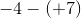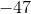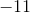Explanation:

When adding with negative values, the sign turns negative. So now we have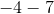. Since they have the same sign, we just treat it as an addition problem and add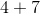and then insert the negative sign in front. The answer is### Example Question #12 : Understand Distances Between Numbers On A Number Line: Ccss.Math.Content.7.Ns.A.1b

Solve: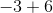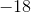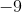When dealing with negative numbers, let's see which number is greater.is greater thanand is positive so the answer is positive. We treat this as a subtraction problem.